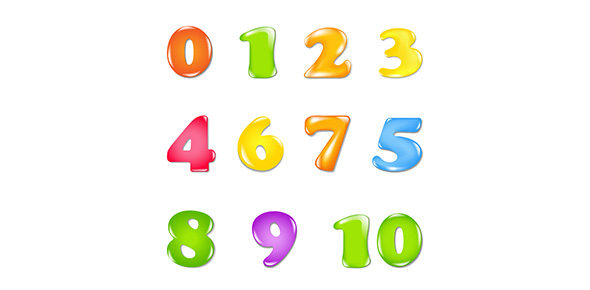# Math Number Quizes

4 Questions | Total Attempts: 56SettingsThis quiz tells you about math. Math is really important so this basically contains numbers.

Related Topics
• 1.
(x+100)(X+60)
• A.

X cubed plus one hundred sixty x plus one hundred sixty

• B.

5

• C.

X plus 45

• D.

a times b times c

• E.

92

• 2.
5 to the second power
• A.

25

• B.

10

• C.

30

• D.

100

• E.

12

• 3.
.5 + .25
• A.

1

• B.

.250

• C.

.30

• D.

1.25

• E.

0

• 4.
(x+5)(x-5)
• A.

X squared minus twenty five

• B.

45x

• C.

75278x

• D.

121x

• E.

45 x squared